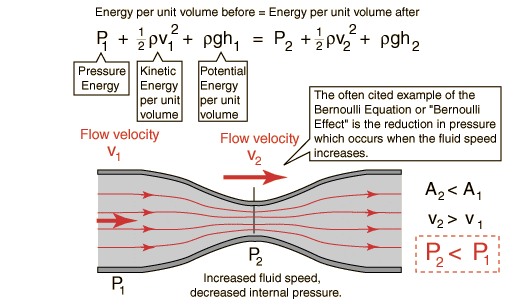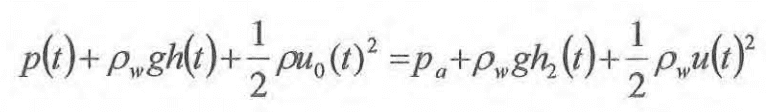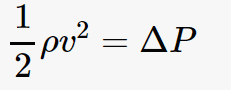# Average water mass flow rate

Hey, I'm a student who has to complete a written assignment for physics. I chose to launch water rockets and investigate the effect of volume on the range. I am trying to also come up with a mathematical model however I am struggling to understand an equation

I found the following equation to calculate the average mass flow rate:

ṁ = A * Cd * √(2ρΔP)
(https://www.palmbeachschools.org/sc.../05/Bottle_Rocket_Calculations_Simplified.pdf)

Where:
ṁ is the mass flow rate
A is the area of the nozzle
Cd is the coefficient of drag
p is the density
and ΔP is the average Pressure acting on the water

Does anyone know where this equation is derived from cause I can't find it anywhere?

Thanks in advance for all replies.

Chestermiller
Mentor
This equation starts out from ##\dot{m}=\rho v A##, where v is the exit velocity. Are you familiar with that equation, which is the same as the volumetric flow rate times the density? If the exit velocity were determined by the Bernoulli equation, then it would satisfy:
$$\frac{1}{2}\rho v^2=\Delta P$$##C_d## is a nozzle discharge factor to correct for nozzle shape and non-Bernoulli deviation. It is typically on the order of unity.

This equation starts out from ##\dot{m}=\rho v A##, where v is the exit velocity. Are you familiar with that equation, which is the same as the volumetric flow rate times the density? If the exit velocity were determined by the Bernoulli equation, then it would satisfy:
$$\frac{1}{2}\rho v^2=\Delta P$$##C_d## is a nozzle discharge factor to correct for nozzle shape and non-Bernoulli deviation. It is typically on the order of unity.

Thanks so much, I now understand. I watched some videos and found this helpful diagram:But I don't see how to get the average pressure(ΔP) from Bernoulli's???

#### Attachments

Chestermiller
Mentor
Thanks so much, I now understand. I watched some videos and found this helpful diagram:
View attachment 229734
But I don't see how to get the average pressure(ΔP) from Bernoulli's???

How do I get from this:to this#### Attachments

haruspex
Homework Helper
Gold Member
haruspex
Homework Helper
Gold Member
ṁ = A * Cd * √(2ρΔP)
The equation makes sense except for the Cd term. In many rocketry equations there is a drag term for the air drag on the rocket, but that would not be relevant here since we are concerned only with the speed of the exhaust, not the acceleration of the rocket. So presumably it represents the nozzle drag on the water flow. But in that case the greater the drag the lower the flow rate, which is not what the equation says.
I would have expected ṁ = A * √(2ρΔP/Cd) (though not necessarily the factor 2).

Chestermiller
Mentor
The equation makes sense except for the Cd term. In many rocketry equations there is a drag term for the air drag on the rocket, but that would not be relevant here since we are concerned only with the speed of the exhaust, not the acceleration of the rocket. So presumably it represents the nozzle drag on the water flow. But in that case the greater the drag the lower the flow rate, which is not what the equation says.
I would have expected ṁ = A * √(2ρΔP/Cd) (though not necessarily the factor 2).
Cd is the discharge coefficient, related to the nozzle shape (Google discharge coefficient). Typically, Cd is < 1, so it does result in reduced discharge mass flow rate.

haruspex
Homework Helper
Gold Member
Cd is the discharge coefficient, related to the nozzle shape (Google discharge coefficient). Typically, Cd is < 1, so it does result in reduced discharge mass flow rate.
Ok, thanks.

Hi guys, pleas can someone run me through exactly how the equation is derived? Up until you end up at
ṁ = A * Cd * √(2ρΔP)

haruspex
Homework Helper
Gold Member
Hi guys, pleas can someone run me through exactly how the equation is derived? Up until you end up at
ṁ = A * Cd * √(2ρΔP)
Can you answer any of my questions in post #6?

Can you answer any of my questions in post #6?
No, unfortunately I cannot. Can you answer mine?

haruspex